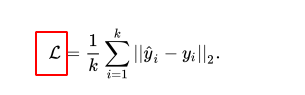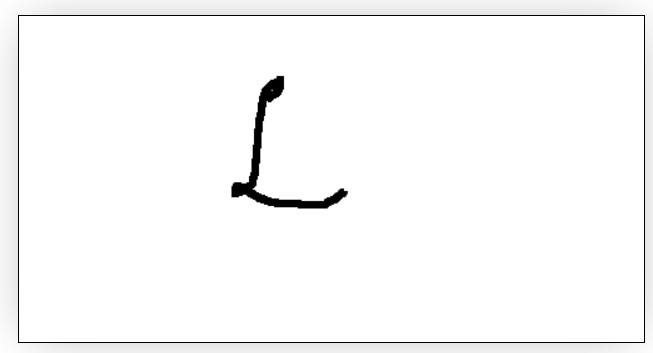# 这个 LaTeX 字符怎么输？\mathcal{L}

1 个赞

\mathcal{L}=\frac{1}{k} \sum_{i=1}^{k}\left|\hat{y}{i}-y{i}\right|_{2}

Mathpix 了解下

2 个赞

http://shapecatcher.com/ 这个手写符号识别网站挺好用的5 个赞